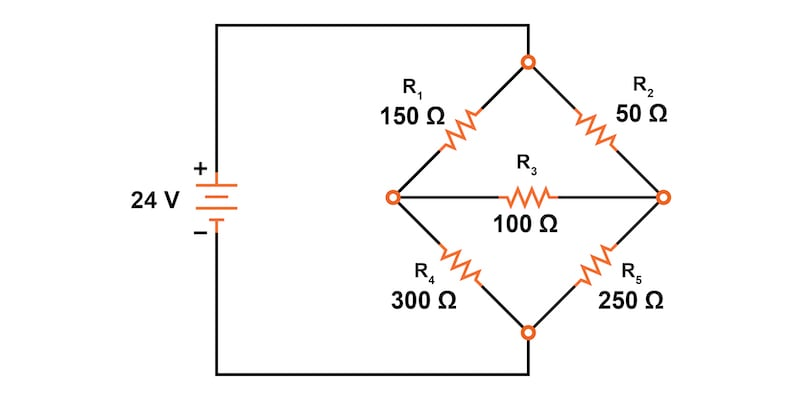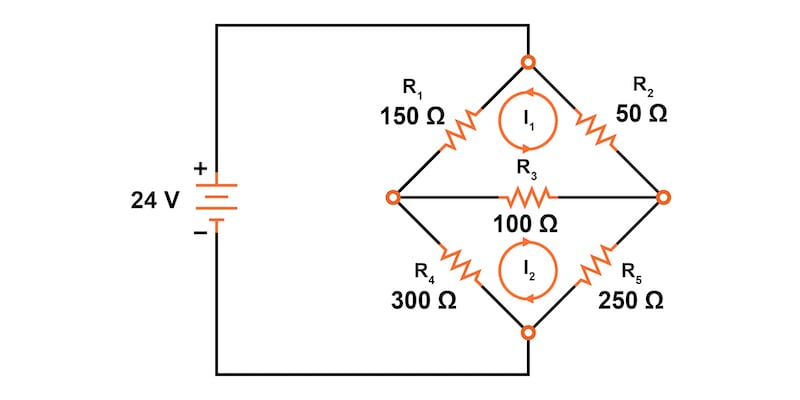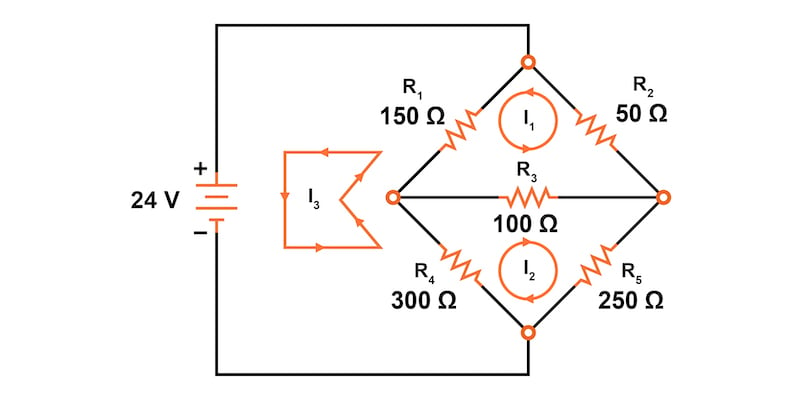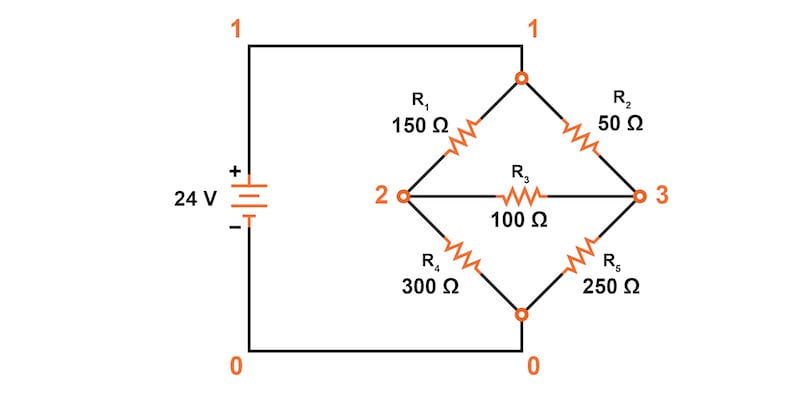Vol. Direct Current (DC)
Chapter 10 DC Network Analysis

# Solving Unbalanced Wheatstone Bridge Circuits Via Mesh Current Method### Using the Mesh Current Method to Solve Unbalanced Wheatstone Bridge Circuits

Solving for the currents and voltages in an unbalanced Wheatstone bridge circuit can be challenging. Learn about solving currents and voltages using the mesh current method.

An unbalanced Wheatstone bridge cannot be solved using simple series and parallel circuit analysis because the resistors are connected in a complex configuration. This section provides a step-by-step walkthrough demonstrating how to solve an unbalanced Wheatstone bridge using the mesh current method (also known as the loop current method).

### Unbalanced Wheatstone Bridge

First, let’s determine the voltages and currents for the unbalanced Wheatstone bridge circuit of Figure 1.##### Figure 1. Unbalanced Wheatstone bridge circuit schematic.

Since the ratios of R/ R4 and R/ R5 are not equal, there will be a voltage across the resistor, R3, and some amount of current through it. As discussed at the beginning of this chapter on network analysis theorems, this type of circuit is irreducible by normal series-parallel analysis and may only be analyzed by some other method.

We could apply the branch current method to this circuit, but it would require six currents (I1 through I6), leading to a large set of simultaneous equations to solve. On the other hand, using the mesh current method, we may solve for all currents and voltages with fewer variables.

Below are the six steps of the mesh current method:

1. Identify and label the current loops
2. Label the voltage drop polarities
3. Apply Kirchhoff’s voltage law (KVL) to each loop
4. Solve the simultaneous equations for the unknown currents
5. Redraw the mesh currents and determine the branch currents
6. Calculate the voltage drops using Ohm’s law

### Step 1: Identify and Label the Current Loops

The first step in the mesh current method is to draw just enough mesh currents to account for all components in the circuit. Looking at our bridge circuit, it should be obvious where to place two of these current loops, as shown in Figure 2.##### Figure 2. Current loops 1 and 2 were added to the Wheatstone bridge circuit.

The directions of these mesh currents, of course, are arbitrary. However, two mesh currents are insufficient in this circuit because neither I1 nor I2 go through the battery. Thus, we must add a third mesh current, I3, as illustrated in Figure 3.##### Figure 3. Current loop 3 added to the Wheatstone bridge circuit.

Here, we’ve chosen I3 to loop from the negative terminal of the battery, through R4 through R1, and back to the positive terminal of the battery. This is one of many paths that could have been chosen for I3, but this example seems the simplest.

### Step 2: Label the Voltage Drop Polarities

Now, we must label the resistor voltage drop polarities, following each current direction (Figure 4).### Step 3: Apply Kirchhoff’s Voltage Law to Each Loop

Next, let’s generate a KVL equation for the top loop of the bridge, starting from the top node and tracing it in a clockwise direction. This results in the following equations:

$$R_2I_1 + R_3(I_1 + I_2) + R_1(I_1 - I_3) = = 0 \text{ V}$$

$$50I_1 +100(I_1 + I_2) + 150(I_1 - I_3) = 0 \text{ V}$$

In this equation, we represent the current through each resistor as the algebraic sum of the loop currents. For example, resistor R3 has its voltage represented in the above KVL equation by the expression 100(I1 + I2) since both currents, I1 and I2, go through R3 in the same direction. The voltage across resistor R1 is expressed as 150(I1 - I3) since loop currents I1 and I3 oppose each other.

Combining like terms, we can simplify this to:

$$300I_1 +100I_2 - 150I_3 = 0 \text{ V}$$

Now, we can repeat that same process to generate a KVL equation for the bottom loop of the Wheatstone bridge. Let’s start at the bottom and move counterclockwise.

$$R_5I_2 + R_3(I_2+I_1) + R_4(I_2+I_3) = = 0 \text{ V}$$

$$250I_2 + 100(I_2+I_1) + 300(I_2+I_3) =0 \text{ V}$$

After combining like terms, we get the following equation:

$$100I_1 + 650I_2 + 300I_3 =0 \text{ V}$$

Note how the second term in the equation’s original form has resistor R3‘s value of 100 Ω multiplied by the sum of I2 and I1 (I2 + I1) because those currents flow in the same direction through R3. The same applies to currents I2 and I3 passing through resistor R4.

With that done, we’ve now taken care of two equations; however, we still need a third equation to complete the simultaneous equation set of three variables and three equations. This third equation must also include the battery’s voltage, which up to this point, does not appear in either two of the previous KVL equations.

To generate this equation, we will trace a loop again with our imaginary voltmeter starting from the battery’s bottom (negative) terminal, stepping clockwise. Again, the direction in which we step is arbitrary and does not need to be the same as the direction of the mesh current in that loop.

$$24 + R_1(I_3 - I_1) + R_4(I_3 + I_2) = = 0 \text{ V}$$

$$24 + 150(I_3 - I_1) + 300(I_3 + I_2) = 0 \text{ V}$$

Again, combining like terms and simplifying the equation, we get:

$$-150I_1 + 300I_2 + 450I_3 = 24 \text{ V}$$

### Step 4: Solve the Simultaneous Equations for Unknown Currents

Now we have three simultaneous equations that we can solve using any method we prefer:

$$300I_1 +100I_2 - 150I_3 = 0 \text{ V}$$

##### KVL of Loop 1

$$100I_1 + 650I_2 + 300I_3 =0 \text{ V}$$

##### KVL of Loop 2

$$-150I_1 + 300I_2 + 450I_3 = 24 \text{ V}$$

##### KVL of Loop 3

First off, we will use GNU Octave, an open-source Matlab clone, to solve these equations. We can enter the resistor coefficients into a matrix, A, between square brackets with column elements separated by commas and rows separated by semicolons.

Next, we can enter the voltages into a column vector, b. The unknown currents: I1, I2, and I3 are calculated by the command: x = A \ b. These currents are contained within the resulting x-column vector.


octave:1>A = [300,100,150;100,650,-300;-150,300,-450]
A =
300  100  150
100  650  -300
-150 300  -450

octave:2> b = [0;0;-24]
b =
0
0
-24

octave:3> x = A\b
x =
-0.093793
0.077241
0.136092


Therefore the values of our loop currents are:

• I1 = -93.793 mA
• I2 = 77.241 mA
• I3 = -136.092 mA

### Step 5: Redraw the Mesh Currents and Determine the Branch Currents

The negative values for currents I1 and I3 indicate that our assumed current directions were in the wrong direction. For I3, it makes intuitive sense because the current would have to flow out of the only power source in our circuit.

Thus, the actual loop current directions and the directions resistor are shown in Figure 5.##### Figure 5. Correcting the loop current directions and determining the branch resistor currents.

From here, we can calculate the branch resistor current values:

$$I_{R1} = I_3 - I_1 = 136.092 - 93.793 = 42.299 \text{ mA}$$

$$I_{R2} = I_1 = 93.793 \text{ mA}$$

$$I_{R3} = I_1 - I_2 = 93.793 - 77.241 = 16.552 \text{ mA}$$

$$I_{R4} = I_3 - I_2 = 136.092 - 77.241 = 58.851 \text{ mA}$$

$$I_{R5} = I_2 = 77.241 \text{ mA}$$

### Step 6: Calculate the Voltage Drops

Finally, we can calculate the voltage drops across each resistor:

$$V_{R1} = I_{R1}R_1 = (0.042299) \cdot (150) = 6.3448 \text{ V}$$

$$V_{R2} = I_{R2}R_5 = (0.093793) \cdot (50) = 4.6897 \text{ V}$$

$$V_{R3} = I_{R3}R_5 = (0.016552) \cdot (100) = 1.6552 \text{ V}$$

$$V_{R4} = I_{R4}R_5 = (0.058851) \cdot (300) = 17.6553 \text{ V}$$

$$V_{R5} = I_{R5}R_5 = (0.077241) \cdot (250) = 19.3103 \text{ V}$$

### Using SPICE to Verify Our Voltage Calculations

Next, to confirm the accuracy of our voltage calculations, we can use a SPICE simulation using the circuit of Figure 6.##### Figure 6. Node number labeling for SPICE simulation.

unbalanced wheatstone bridge
v1 1 0
r1 1 2 150
r2 1 3 50
r3 2 3 100
r4 2 0 300
r5 3 0 250
.dc v1 24 24 1
.print dc v(1,2) v(1,3) v(3,2) v(2,0) v(3,0)
.end
v1          v(1,2)     v(1,3)      v(3,2)     v(2)       v(3)
2.400E+01   6.345E+00  4.690E+00   1.655E+00  1.766E+01  1.931E+01


### Mesh Current Method for an Unbalanced Wheatstone Bridge Review

Using the mesh current method, we were able to solve for the currents and voltages in an unbalanced Wheatstone bridge. The three simultaneous KVL equations with three unknown loop currents were solved using GNU Octave. We then validated our results using SPICE circuit simulation software.

### Related Content

Below you can find additional resources concerning mesh current analysis and Wheatstone bridge circuits:

Calculators:

Worksheets:

Video Tutorials and Lectures:

Technical Articles: